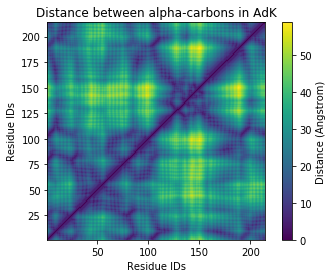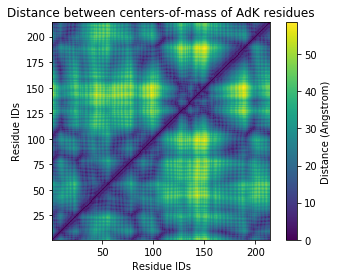# All distances within a selection¶

Here we calculate the distance of every atom to every other atom in a selection, and show how we can extend this to residues.

Last executed: Feb 06, 2020 with MDAnalysis 0.20.2-dev0

Last updated: January 2020

Minimum version of MDAnalysis: 0.19.0

Packages required:

Optional packages for visualisation:

:

import MDAnalysis as mda
from MDAnalysis.tests.datafiles import PDB_small
from MDAnalysis.analysis import distances

import numpy as np
import matplotlib.pyplot as plt
%matplotlib inline


The test files we will be working with here feature adenylate kinase (AdK), a phosophotransferase enzyme. ([BDPW09])

:

u = mda.Universe(PDB_small)


## Calculating atom-wise distances¶

We begin by selecting the alpha-carbons of the protein.

:

ca = u.select_atoms('name CA')
n_ca = len(ca)
n_ca

:

214


When given an array with $$n$$ positions, distances.self_distance_array returns the distances in a flat vector with length $$\frac{n(n-1)}{2}$$. These correspond to the flattened upper triangular values of a square distance matrix.

:

self_distances = distances.self_distance_array(ca.positions)
self_distances.shape

:

(22791,)


We can convert this into a more easily interpreted square distance array. First we create an all-zero square array and get the indices of the upper and lower triangular matrices.

:

sq_dist_arr = np.zeros((n_ca, n_ca))
triu = np.triu_indices_from(sq_dist_arr, k=1)


Then we simply assign the calculated distances to the upper and lower triangular positions.

:

sq_dist_arr[triu] = self_distances
sq_dist_arr.T[triu] = self_distances


### Plotting¶

:

fig, ax = plt.subplots()
im = ax.pcolor(ca.resids, ca.resids, sq_dist_arr)

# plt.pcolor gives a rectangular grid by default
# so we need to make our heatmap square
ax.set_aspect('equal')

# add figure labels and titles
plt.ylabel('Residue IDs')
plt.xlabel('Residue IDs')

# colorbar
cbar = fig.colorbar(im)
cbar.ax.set_ylabel('Distance (Angstrom)')

:

Text(0, 0.5, 'Distance (Angstrom)')## Calculating distances for each residue¶

Instead of calculating the distance between the alpha-carbon of each residue, we could look at the distances between the centers-of-mass instead. The process is very similar to the atom-wise distances above, but we have to pass distances.self_distance_array an array of residue center-of-mass coordinates instead.

:

res_com = u.atoms.center_of_mass(compound='residues')
n_res = len(res_com)
n_res

:

214


As the number of residues remains the same, the resulting distances array has the same length.

:

res_dist = distances.self_distance_array(res_com)
res_dist.shape

:

(22791,)


This means we don’t need to re-define triu.

:

sq_dist_res = np.zeros((n_res, n_res))
sq_dist_res[triu] = res_dist
sq_dist_res.T[triu] = res_dist


### Plotting¶

The resulting plot looks pretty similar.

:

fig2, ax2 = plt.subplots()
im2 = ax2.pcolor(u.residues.resids, u.residues.resids, sq_dist_res)

# plt.pcolor gives a rectangular grid by default
# so we need to make our heatmap square
ax2.set_aspect('equal')

# add figure labels and titles
plt.ylabel('Residue IDs')
plt.xlabel('Residue IDs')
plt.title('Distance between centers-of-mass of AdK residues')

# colorbar
cbar2 = fig2.colorbar(im2)
cbar2.ax.set_ylabel('Distance (Angstrom)')

:

Text(0, 0.5, 'Distance (Angstrom)')